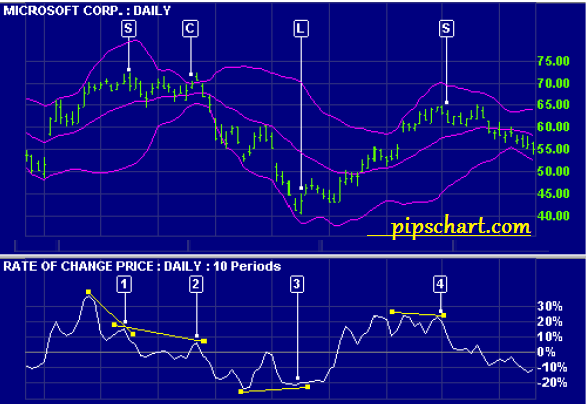# Relative Strength Index (RSI) Only Use 14 Periods (why?)

1,796 views

## RSI Only Use 14 Periods – Why?

RSI only use 14 periods why? All known it but no one find why? In Forex trading RSI play a vital role for successful trading.let fine RSI. RSI – Relative Strength Indicator is developed by J. Welles Wilder and introduced in his book, New Concepts in Technical Trading Systems, the Relative Strength Index (RSI) is an extremely popular momentum oscillator.

The RSI compares the magnitude of a stock’s recent gains to the magnitude of its recent losses and turns that information into a number that ranges from 0 to 100. It takes a single parameter, the number of time periods to use in the calculation. Wilder recommends using 14 periods.

The RSI’s full name is actually rather unfortunate as it is easily confused with other forms of Relative Strength analysis. Most other kinds of “Relative Strength” stuff involve using more than one stock in the calculation.

Like most true indicators, the RSI only needs one stock to be computed. In order to avoid confusion, avoid using the RSI’s full name and just call it “the RSI.”

To be used in conjunction with Bollinger Bands, the Relative Strength Indicator or index is based on a ratio of the average upward changes to the average downward changes over a given period of time. It has a range of 0 to 100 with values typically remaining between 30 and 70.

Higher values indicate overbought conditions while lower values indicate oversold conditions.The Relative Strength Index at the beginning of a data series is not defined until there are enough values to fill the given period. In addition, the value is defined as 100 when no downward changes occur during the given period.

The Relative Strength Index (RSI) is typically used with a 9, 14, or 25 calendar day (7, 10, or 20 trading day) period against the closing price of a security or commodity. The more days that are included in the calculation, the less volatile the value. The RSI usually leads the price by forming peaks and valleys before the price data, especially around the values of 30 and 70.

In addition, when the RSI diverges from the price, the price will eventually correct to the direction of the index. The Relative Strength Index function determines the internal strength of a field using the number of upward and downward price changes over a given period of time. To simplify the formula, the RSI has been broken down into its basic components which are the Average Gain, the Average Loss, the First RS, and the subsequent Smoothed RS’s.

For a 14-period RSI, the Average Gain equals the sum total all gains divided by 14. Even if there are only 5 gains (losses), the total of those 5 gains (losses) is divided by the total number of RSI periods in the calculation (14 in this case).

The Average Loss is computed in a similar manner. Calculation of the First RS value is straightforward: divide the Average Gain by the Average Loss. All subsequent  RS calculations use the previous period’s Average Gain and Average Loss for smoothing purposes.

When the Average Gain is greater than the Average Loss, the RSI rises because RS will be greater than 1. Conversely, when the average loss is greater than the average gain, the RSI declines because RS will be less than 1. The last part of the formula ensures that the indicator oscillates between 0 and 100. Wilder recommended using 70 and 30 and overbought and oversold levels respectively.

Generally, if the RSI rises above 30 it is considered bullish for the underlying stock. Conversely, if the RSI falls below 70, it is a bearish signal. Some traders identify the long-term trend and then use extreme readings for entry points. If the long-term trend is bullish, then oversold readings could mark potential entry points.Buy and sell signals can also be generated by looking for positive and negative divergences between the RSI and the underlying stock. For example, consider a falling stock whose RSI rises from a low point of (for example) 15 back up to say, 55.

Because of how the RSI is constructed, the underlying stock will often reverse its direction soon after such a divergence. As in that example, divergences that occur after an overbought or oversold reading usually provide more reliable signals.

The center line for RSI is 50. Readings above and below can give the indicator a bullish or bearish tilt. On the whole, a reading above 50 indicates that average gains are higher than average losses and a reading below 50 indicates that losses are winning the battle. Some traders look for a move above 50 to confirm bullish signals or a move below 50 to confirm bearish signals.

Happy trading……………………..

Relative Strength Index (RSI) Only Use 14 Periods (why?)
5 (100%) 1 vote[s]